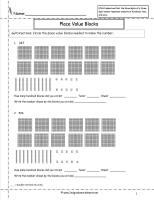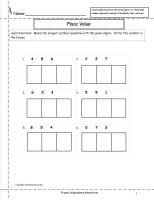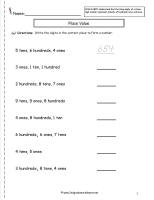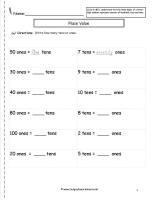﻿  CCSS 2.NBT.1 Worksheets Place Value Worksheets.

# Common Core State Standard 2.NBT.1

## Number & Operations in Base Ten

### Understand Place Value.

1. Understand that the three digits of a three-digit number represent amounts of hundreds, tens, and ones; e.g., 706 equals 7 hundreds, 0 tens, and 6 ones. Understand the following as special cases:

a.  100 can be thought of as a bundle of ten tens — called a “hundred.”
b.  The numbers 100, 200, 300, 400, 500, 600, 700, 800, 900 refer to one, two, three, four, five, six, seven, eight, or nine hundreds (and 0 tens and 0 ones).

###Place Value Worksheet  12345678910

Common Core State Standards: 2.NBT.1
Numbers & Operations in Base Ten
Understand Place Value

Understand that the three digits of a three-digit number represent amounts of hundreds, tens, and ones; e.g., 706 equals 7 hundreds, 0 tens, and 6 ones. Understand the following as special cases:

Students circle place value blocks to make a number.

###Place Value Worksheet  12

Common Core State Standards: 2.NBT.1
Numbers & Operations in Base Ten
Understand Place Value

Understand that the three digits of a three-digit number represent amounts of hundreds, tens, and ones; e.g., 706 equals 7 hundreds, 0 tens, and 6 ones. Understand the following as special cases:

Students circle the place value blocks that represent the underlined digit.

###Place Value Worksheet  12 Number Cards

Common Core State Standards: 2.NBT.1
Numbers & Operations in Base Ten
Understand Place Value

Understand that the three digits of a three-digit number represent amounts of hundreds, tens, and ones; e.g., 706 equals 7 hundreds, 0 tens, and 6 ones. Understand the following as special cases:

Students make the biggest number possible with given digits.

###Biggest Number Game

Common Core State Standards: 2.NBT.1
Numbers & Operations in Base Ten
Understand Place Value

Understand that the three digits of a three-digit number represent amounts of hundreds, tens, and ones; e.g., 706 equals 7 hundreds, 0 tens, and 6 ones. Understand the following as special cases:

Students make the biggest number possible with given digits.

###Place Value Worksheet2345

Common Core State Standards: 2.NBT.1
Numbers & Operations in Base Ten
Understand Place Value

Understand that the three digits of a three-digit number represent amounts of hundreds, tens, and ones; e.g., 706 equals 7 hundreds, 0 tens, and 6 ones. Understand the following as special cases:

Students write how many hundred, tens, or ones are shown.

###Place Value Worksheet  12

Common Core State Standards: 2.NBT.1
Numbers & Operations in Base Ten
Understand Place Value

Understand that the three digits of a three-digit number represent amounts of hundreds, tens, and ones; e.g., 706 equals 7 hundreds, 0 tens, and 6 ones. Understand the following as special cases:

Students write the correct number shown by the digits and places.

###Place Value Worksheet  12

Common Core State Standards: 2.NBT.1
Numbers & Operations in Base Ten
Understand Place Value

Understand that the three digits of a three-digit number represent amounts of hundreds, tens, and ones; e.g., 706 equals 7 hundreds, 0 tens, and 6 ones. Understand the following as special cases: